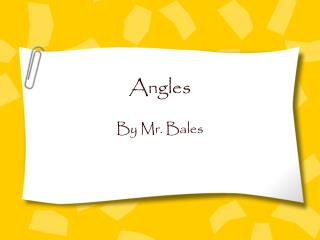DownloadDownload PresentationAngles

# Angles

Télécharger la présentation## Angles

- - - - - - - - - - - - - - - - - - - - - - - - - - - E N D - - - - - - - - - - - - - - - - - - - - - - - - - - -
##### Presentation Transcript

1. Angles By Mr. Bales

2. Objective • By the end of this lesson, you will be able to identify, describe, and classify angles. • Standard 4MG3.5 - Students need to know the definitions of a right angle, an acute angle, and an obtuse angle. Understand that 90°, 180°, 270°, and 360° are associated respectively, with 1/4, 1/2, 3/4, and full turns.

3. Turns • How many degrees does it take to go in a complete circle? Or make one full turn? • Let’s watch a little NBA to find out!! • NBA • So, how many degrees are there in a full turn?

4. History Lesson • The Babylonians in about 2400 B.C. lived in Mesopotamia, now southern Iraq. They used a number system based on 60. Some believe they divided the circle into 360 degrees because 6 x 60 = 360 degrees. Others believed that they watched the planets and the sun. They knew it took 360 days for the sun’s path to complete a total circuit. Either way, that is how we got the 360 degree circle.

5. Get Ready to Learn • MJ’s teacher (that’s me) assigned ten problems for homework. MJ started his homework at 4:00 p.m. He completed it at 4:15 p.m. How far has the minute hand turned? • Use the following pictures to help you solve the problem.

6. 270° More on Turns 1/2 turn ¼ turn 90° 180° full turn ¾ turn 360°

7. Activity • Let’s get on our feet and practice this!! • Please stand and face the board, which is 12:00. • Turn 1/4 turn. • What angle measure is that? • Turn 1/2 turn. • What angle measure is that? • Turn 3/4 turn. • What angle measure is that? • Turn a full turn. • What angle measure is that?

8. A How would you label this Daniella? B C What are Angles? • Anglesare figures that are formed by two rays or two line segments with a common endpoint. • You label angles with a < symbol and you place the vertex in the middle of the three points. Perfect! < ABC or < CBA Always label angles with vertex in the middle!

9. S Can you label this correctly? T U Right Angles • There are all kinds of different angles. • A right angle has a “square corner”.

10. Acute Angles • An acute angle measures greater than 0º and less than 90º.

11. Obtuse Angles • An obtuse angle measures greater than 90º, but less than 180º.

12. More on Angles • Let’s hear from Mr. Hardesty as he explains a few different angles. • Mr. Hardesty!!

13. Bales Bucks!!!

14. Assignment • Page 400 - #’s 8 - 19 • Get them all correct, No Math Homework